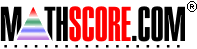Math Practice Online > free > lessons > Florida > 6th grade > Triangle Angles

## Triangle Angles

Utilize the fact that the sum of angles in a triangle is 180. You may need pencil and paper.

 Sample Problems for Triangle Angles Lesson for Triangle Angles

### This topic aligns to the following state standards

Grade 6: Geo 6. Knows the properties of two- and three-dimensional figures.
Grade 7: Geo 6. Knows the properties of two- and three-dimensional figures.
Grade 8: Geo 1. Determines and justifies the measures of various types of angles based upon geometric relationships in two- and three-dimensional shapes.
Grade 8: Geo 4. Knows the properties of two- and three-dimensional figures.
Grade 7: Geo 2. Creates and solves angle measurement problems for triangles.

Copyright Accurate Learning Systems Corporation 2008.